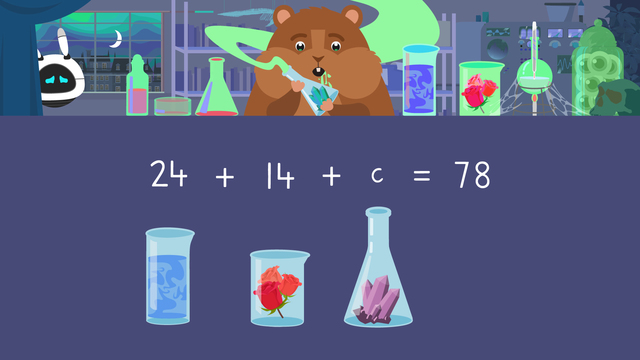# Equations with Addition and SubtractionRate this video

Ø 5.0 / 3 ratings

The authorTeam Digital
CCSS.MATH.CONTENT.4.OA.A.3

### Contents

In this video, Mr. Squeaks just finished an experiment that has gone wrong! He has turned himself into the evil Dr. Squeaks! Imani has to follow the instructions to turn him back. Let’s help Imani by learning how to solve equations with addition and subtraction!

### Equations with Addition and Subtraction

#### What is an Equation?

An equation tells us that the expression on either side of the equal sign is equal to the other. Sometimes, when writing an equation, you may have an unknown value that you need to solve for. A variable is a letter used to represent the value that we're solving for.

Equations can be used to solve word problems by following these steps:

• Step 1: Read the word problem
• Step 2: Highlight the important information
• Step 3: Identify the operation
• Step 4: Write the equation using a variable to represent the unknown value
• Step 5: Solve the equation

### How to Solve Equations Using Addition and Subtraction

How do you solve addition and subtraction equations? Let’s take a look at the example from the video:

In order to reverse the experiment, Imani needs seventy-eight ingredients altogether. The directions say they need twenty-four jars of mist and fourteen rose petals. The rest of the ingredients they need are crystals. How many crystals does Imani need?Our equation is: twenty-four jars of mist plus fourteen rose petals plus c equals seventy-eight because if we add all the ingredients together, the total number they need is seventy-eight.

#### Let’s Solve

First, we add twenty four jars of mist and fourteen rose petals to get thirty-eight, but we still don't know how many crystals we need!

This is an addition equation, but since we do not know the value of c, we need to practice solving an addition equation using inverse operations.The inverse operation of addition is subtraction, so we subtract thirty-eight from the number of ingredients in total, which is seventy-eight. Seventy-eight minus thirty-eight is forty, and we write the answer using the variable c equals forty.### Equations with Addition and Subtraction Summary

Remember an equation tells us that the expression on either side of the equal sign is EQUAL to the other. Equations can be used to solve word problems. When writing the equation we use a variable to represent the unknown value. Sometimes when solving equations with addition and subtraction you may have to use the inverse operation to find the answer. Always include the variable in your answer using an equal sign.

Want some more solving equations with addition and subtraction practice? On this website there is a solving equations with addition and subtraction worksheet along with other activities, and exercises.

### TranscriptEquations with Addition and Subtraction

Transkripte - DACH Prozess Transkripte - DACH Prozess 100% 10 C2:J100